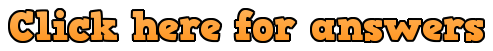Workout

Question 1

(a)  x = 1, x = 3               (b)   y = 4, y = 9               (c) m = −1, m = −6

(d)  x = 3, x = −2            (e)  t = −7, t = 3               (f) k = 10, k = −9

(g)  w = −5, w = −11       (h)  y = 8, y = 2               (i)  x = −3, x = 9

Question 2

(a)  x = −4, x = −2         (b)  x = −4, x = −3            (c)  y = −5,  y= −2

(d)  y = −4, y = 1            (e)  x = −2, x = 4              (f)  m = 3, m = 4

(g)  y = 5                          (h)  y = −5, y = 9              (i)  x = −7, x = 8

(j)  y = −6, y = −4          (k)  x = −6, x = −3            (l)  x = −22, x = −1

(m)  y = 2, y = 11           (n)  x = −4, x = 3              (o)  m = −3, m = 9

(p) x = 2, x = 9               (q)  y = 6, y = 8                (r)  x = 7, x = 8

(s)  m = −7, m = 8         (t)  y = −16, y = −6          (u)  k = −4, k = 22

(v)  x = 2, x = 36            (w)  x = −17, x = 3            (x)  y = −20, y = −12

(y)  g = −4, g = 16          (z)  y = −11

Question 3

(a)  y = −5, y = 5             (b)  x = −2, x = 2                (c)  m = −9, m = 9

Question 4

(a)  x = −3, x = 3             (b)  y = −10, y = 10            (c) w = −1, w = 1

(d) k = −12, k = 12          (e)  x = −8, x = 8                (f) c = −0.5, c = 0.5

Question 5

(a)  x = −1                         (b)  y = −7, y = −1             (c)  y = 3, y = 4

(d)  y = −12, y = −1         (e)  x = −2, x = 5                (f)  x = 7

(g)  x = 3                           (h)  x = −14, x = 15

Question 6

(a)  x = 1, x = 5                (b)  x = −5, x = 3                 (c)  x = −9, x = 5

Apply

Question 1:

(a)  x² − 3x − 180 = 0

(b)  Alex is 15

Question 2:  180m

Question 3:  height = 17cm, base = 10cm

Question 4:  15 and 12

Question 5:  36cm

Question 6:

(a)  x² + x² + (x² + 15x) + (x² + 15x) + (x² + 15x) + (x² + 15x)

6x² + 60x = 3600

x² + 10x = 600

x² + 10x − 600 = 0

(b)  x = 20

(c)  14000cm³

Question 7:  x = 7  or x = 11

Question 8: